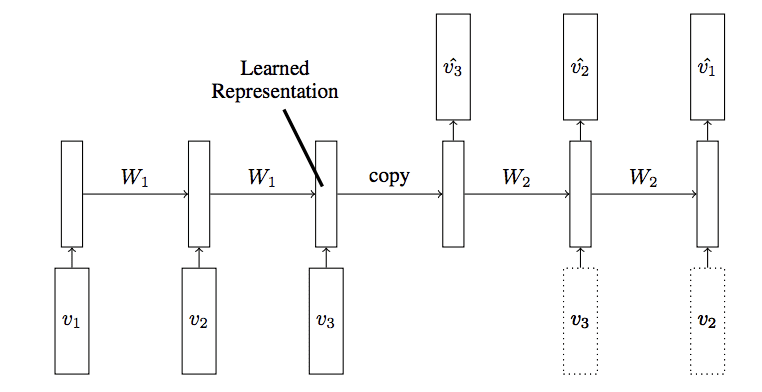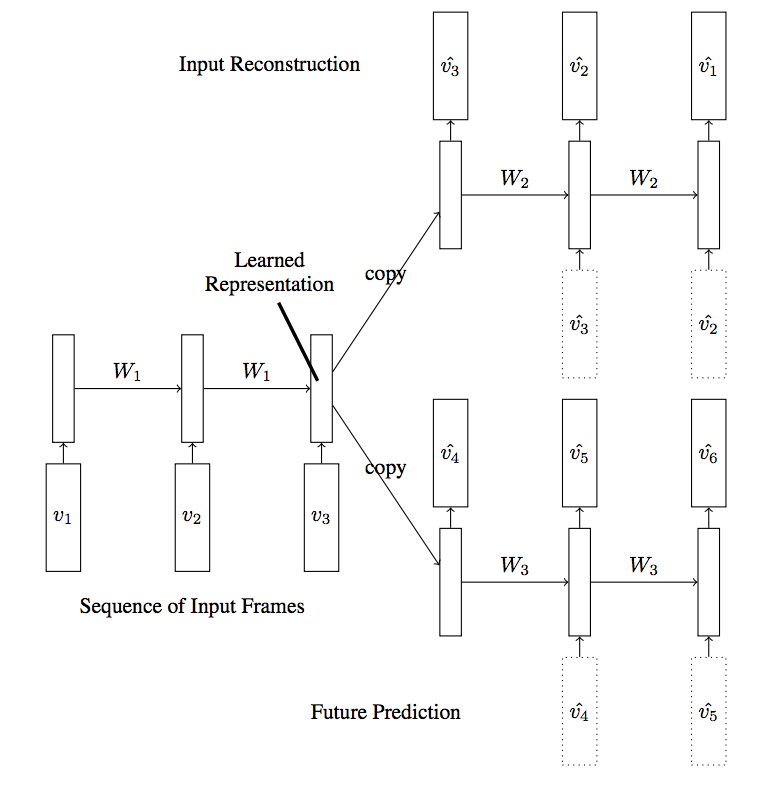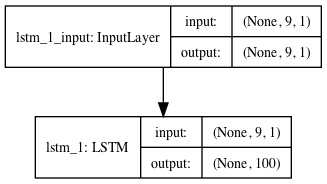Data Science

# A Gentle Introduction to LSTM Autoencoders

An LSTM Autoencoder is an implementation of an autoencoder for sequence data using an Encoder-Decoder LSTM architecture.

Once fit, the encoder part of the model can be used to encode or compress sequence data that in turn may be used in data visualizations or as a feature vector input to a supervised learning model.

In this post, you will discover the LSTM Autoencoder model and how to implement it in Python using Keras.

After reading this post, you will know:

• Autoencoders are a type of self-supervised learning model that can learn a compressed representation of input data.
• LSTM Autoencoders can learn a compressed representation of sequence data and have been used on video, text, audio, and time series sequence data.
• How to develop LSTM Autoencoder models in Python using the Keras deep learning library.

Let’s get started.A Gentle Introduction to LSTM Autoencoders
Photo by Ken Lund, some rights reserved.

## Overview

This post is divided into six sections; they are:

1. What Are Autoencoders?
2. A Problem with Sequences
3. Encoder-Decoder LSTM Models
4. What Is an LSTM Autoencoder?
5. Early Application of LSTM Autoencoder
6. How to Create LSTM Autoencoders in Keras

## What Are Autoencoders?

An autoencoder is a neural network model that seeks to learn a compressed representation of an input.

They are an unsupervised learning method, although technically, they are trained using supervised learning methods, referred to as self-supervised. They are typically trained as part of a broader model that attempts to recreate the input.

For example:

`X = model.predict(X)`

The design of the autoencoder model purposefully makes this challenging by restricting the architecture to a bottleneck at the midpoint of the model, from which the reconstruction of the input data is performed.

There are many types of autoencoders, and their use varies, but perhaps the more common use is as a learned or automatic feature extraction model.

In this case, once the model is fit, the reconstruction aspect of the model can be discarded and the model up to the point of the bottleneck can be used. The output of the model at the bottleneck is a fixed length vector that provides a compressed representation of the input data.

Input data from the domain can then be provided to the model and the output of the model at the bottleneck can be used as a feature vector in a supervised learning model, for visualization, or more generally for dimensionality reduction.

## A Problem with Sequences

Sequence prediction problems are challenging, not least because the length of the input sequence can vary.

This is challenging because machine learning algorithms, and neural networks in particular, are designed to work with fixed length inputs.

Another challenge with sequence data is that the temporal ordering of the observations can make it challenging to extract features suitable for use as input to supervised learning models, often requiring deep expertise in the domain or in the field of signal processing.

Finally, many predictive modeling problems involving sequences require a prediction that itself is also a sequence. These are called sequence-to-sequence, or seq2seq, prediction problems.

You can learn more about sequence prediction problems here:

## Encoder-Decoder LSTM Models

Recurrent neural networks, such as the Long Short-Term Memory, or LSTM, network are specifically designed to support sequences of input data.

They are capable of learning the complex dynamics within the temporal ordering of input sequences as well as use an internal memory to remember or use information across long input sequences.

The LSTM network can be organized into an architecture called the Encoder-Decoder LSTM that allows the model to be used to both support variable length input sequences and to predict or output variable length output sequences.

This architecture is the basis for many advances in complex sequence prediction problems such as speech recognition and text translation.

In this architecture, an encoder LSTM model reads the input sequence step-by-step. After reading in the entire input sequence, the hidden state or output of this model represents an internal learned representation of the entire input sequence as a fixed-length vector. This vector is then provided as an input to the decoder model that interprets it as each step in the output sequence is generated.

You can learn more about the encoder-decoder architecture here:

## What Is an LSTM Autoencoder?

An LSTM Autoencoder is an implementation of an autoencoder for sequence data using an Encoder-Decoder LSTM architecture.

For a given dataset of sequences, an encoder-decoder LSTM is configured to read the input sequence, encode it, decode it, and recreate it. The performance of the model is evaluated based on the model’s ability to recreate the input sequence.

Once the model achieves a desired level of performance recreating the sequence, the decoder part of the model may be removed, leaving just the encoder model. This model can then be used to encode input sequences to a fixed-length vector.

The resulting vectors can then be used in a variety of applications, not least as a compressed representation of the sequence as an input to another supervised learning model.

## Early Application of LSTM Autoencoder

One of the early and widely cited applications of the LSTM Autoencoder was in the 2015 paper titled “Unsupervised Learning of Video Representations using LSTMs.”LSTM Autoencoder Model
Taken from “Unsupervised Learning of Video Representations using LSTMs”

In the paper, Nitish Srivastava, et al. describe the LSTM Autoencoder as an extension or application of the Encoder-Decoder LSTM.

They use the model with video input data to both reconstruct sequences of frames of video as well as to predict frames of video, both of which are described as an unsupervised learning task.

The input to the model is a sequence of vectors (image patches or features). The encoder LSTM reads in this sequence. After the last input has been read, the decoder LSTM takes over and outputs a prediction for the target sequence.

More than simply using the model directly, the authors explore some interesting architecture choices that may help inform future applications of the model.

They designed the model in such a way as to recreate the target sequence of video frames in reverse order, claiming that it makes the optimization problem solved by the model more tractable.

The target sequence is same as the input sequence, but in reverse order. Reversing the target sequence makes the optimization easier because the model can get off the ground by looking at low range correlations.

They also explore two approaches to training the decoder model, specifically a version conditioned in the previous output generated by the decoder, and another without any such conditioning.

The decoder can be of two kinds – conditional or unconditioned. A conditional decoder receives the last generated output frame as input […]. An unconditioned decoder does not receive that input.

A more elaborate autoencoder model was also explored where two decoder models were used for the one encoder: one to predict the next frame in the sequence and one to reconstruct frames in the sequence, referred to as a composite model.

… reconstructing the input and predicting the future can be combined to create a composite […]. Here the encoder LSTM is asked to come up with a state from which we can both predict the next few frames as well as reconstruct the input.LSTM Autoencoder Model With Two Decoders
Taken from “Unsupervised Learning of Video Representations using LSTMs”

The models were evaluated in many ways, including using encoder to seed a classifier. It appears that rather than using the output of the encoder as an input for classification, they chose to seed a standalone LSTM classifier with the weights of the encoder model directly. This is surprising given the complication of the implementation.

We initialize an LSTM classifier with the weights learned by the encoder LSTM from this model.

The composite model without conditioning on the decoder was found to perform the best in their experiments.

The best performing model was the Composite Model that combined an autoencoder and a future predictor. The conditional variants did not give any significant improvements in terms of classification accuracy after fine-tuning, however they did give slightly lower prediction errors.

Many other applications of the LSTM Autoencoder have been demonstrated, not least with sequences of text, audio data and time series.

## How to Create LSTM Autoencoders in Keras

Creating an LSTM Autoencoder in Keras can be achieved by implementing an Encoder-Decoder LSTM architecture and configuring the model to recreate the input sequence.

Let’s look at a few examples to make this concrete.

### Reconstruction LSTM Autoencoder

The simplest LSTM autoencoder is one that learns to reconstruct each input sequence.

For these demonstrations, we will use a dataset of one sample of nine time steps and one feature:

`[0.1, 0.2, 0.3, 0.4, 0.5, 0.6, 0.7, 0.8, 0.9]`

We can start-off by defining the sequence and reshaping it into the preferred shape of [samples, timesteps, features].

```# define input sequence
sequence = array([0.1, 0.2, 0.3, 0.4, 0.5, 0.6, 0.7, 0.8, 0.9])
# reshape input into [samples, timesteps, features]
n_in = len(sequence)
sequence = sequence.reshape((1, n_in, 1))```

Next, we can define the encoder-decoder LSTM architecture that expects input sequences with nine time steps and one feature and outputs a sequence with nine time steps and one feature.

```# define model
model = Sequential()
model.add(LSTM(100, activation='relu', input_shape=(n_in,1)))
model.add(RepeatVector(n_in))
model.add(LSTM(100, activation='relu', return_sequences=True))
model.add(TimeDistributed(Dense(1)))
model.compile(optimizer='adam', loss='mse')```

Next, we can fit the model on our contrived dataset.

```# fit model
model.fit(sequence, sequence, epochs=300, verbose=0)```

The complete example is listed below.

The configuration of the model, such as the number of units and training epochs, was completely arbitrary.

```# lstm autoencoder recreate sequence
from numpy import array
from keras.models import Sequential
from keras.layers import LSTM
from keras.layers import Dense
from keras.layers import RepeatVector
from keras.layers import TimeDistributed
from keras.utils import plot_model
# define input sequence
sequence = array([0.1, 0.2, 0.3, 0.4, 0.5, 0.6, 0.7, 0.8, 0.9])
# reshape input into [samples, timesteps, features]
n_in = len(sequence)
sequence = sequence.reshape((1, n_in, 1))
# define model
model = Sequential()
model.add(LSTM(100, activation='relu', input_shape=(n_in,1)))
model.add(RepeatVector(n_in))
model.add(LSTM(100, activation='relu', return_sequences=True))
model.add(TimeDistributed(Dense(1)))
model.compile(optimizer='adam', loss='mse')
# fit model
model.fit(sequence, sequence, epochs=300, verbose=0)
plot_model(model, show_shapes=True, to_file='reconstruct_lstm_autoencoder.png')
# demonstrate recreation
yhat = model.predict(sequence, verbose=0)
print(yhat[0,:,0])```

Running the example fits the autoencoder and prints the reconstructed input sequence.

The results are close enough, with very minor rounding errors.

```[0.10398503 0.20047213 0.29905337 0.3989646 0.4994707 0.60005534
0.70039135 0.80031013 0.8997728 ]```

A plot of the architecture is created for reference.LSTM Autoencoder for Sequence Reconstruction

### Prediction LSTM Autoencoder

We can modify the reconstruction LSTM Autoencoder to instead predict the next step in the sequence.

In the case of our small contrived problem, we expect the output to be the sequence:

`[0.2, 0.3, 0.4, 0.5, 0.6, 0.7, 0.8, 0.9]`

This means that the model will expect each input sequence to have nine time steps and the output sequence to have eight time steps.

```# reshape input into [samples, timesteps, features]
n_in = len(seq_in)
seq_in = seq_in.reshape((1, n_in, 1))
# prepare output sequence
seq_out = seq_in[:, 1:, :]
n_out = n_in - 1```

The complete example is listed below.

```# lstm autoencoder predict sequence
from numpy import array
from keras.models import Sequential
from keras.layers import LSTM
from keras.layers import Dense
from keras.layers import RepeatVector
from keras.layers import TimeDistributed
from keras.utils import plot_model
# define input sequence
seq_in = array([0.1, 0.2, 0.3, 0.4, 0.5, 0.6, 0.7, 0.8, 0.9])
# reshape input into [samples, timesteps, features]
n_in = len(seq_in)
seq_in = seq_in.reshape((1, n_in, 1))
# prepare output sequence
seq_out = seq_in[:, 1:, :]
n_out = n_in - 1
# define model
model = Sequential()
model.add(LSTM(100, activation='relu', input_shape=(n_in,1)))
model.add(RepeatVector(n_out))
model.add(LSTM(100, activation='relu', return_sequences=True))
model.add(TimeDistributed(Dense(1)))
model.compile(optimizer='adam', loss='mse')
plot_model(model, show_shapes=True, to_file='predict_lstm_autoencoder.png')
# fit model
model.fit(seq_in, seq_out, epochs=300, verbose=0)
# demonstrate prediction
yhat = model.predict(seq_in, verbose=0)
print(yhat[0,:,0])```

Running the example prints the output sequence that predicts the next time step for each input time step.

We can see that the model is accurate, barring some minor rounding errors.

```[0.1657285 0.28903174 0.40304852 0.5096578 0.6104322 0.70671254
0.7997272 0.8904342 ]```

A plot of the architecture is created for reference.LSTM Autoencoder for Sequence Prediction

### Composite LSTM Autoencoder

Finally, we can create a composite LSTM Autoencoder that has a single encoder and two decoders, one for reconstruction and one for prediction.

We can implement this multi-output model in Keras using the functional API. You can learn more about the functional API in this post:

First, the encoder is defined.

```# define encoder
visible = Input(shape=(n_in,1))
encoder = LSTM(100, activation='relu')(visible)```

Then the first decoder that is used for reconstruction.

```# define reconstruct decoder
decoder1 = RepeatVector(n_in)(encoder)
decoder1 = LSTM(100, activation='relu', return_sequences=True)(decoder1)
decoder1 = TimeDistributed(Dense(1))(decoder1)```

Then the second decoder that is used for prediction.

```# define predict decoder
decoder2 = RepeatVector(n_out)(encoder)
decoder2 = LSTM(100, activation='relu', return_sequences=True)(decoder2)
decoder2 = TimeDistributed(Dense(1))(decoder2)```

We then tie the whole model together.

```# tie it together
model = Model(inputs=visible, outputs=[decoder1, decoder2])```

The complete example is listed below.

```# lstm autoencoder reconstruct and predict sequence
from numpy import array
from keras.models import Model
from keras.layers import Input
from keras.layers import LSTM
from keras.layers import Dense
from keras.layers import RepeatVector
from keras.layers import TimeDistributed
from keras.utils import plot_model
# define input sequence
seq_in = array([0.1, 0.2, 0.3, 0.4, 0.5, 0.6, 0.7, 0.8, 0.9])
# reshape input into [samples, timesteps, features]
n_in = len(seq_in)
seq_in = seq_in.reshape((1, n_in, 1))
# prepare output sequence
seq_out = seq_in[:, 1:, :]
n_out = n_in - 1
# define encoder
visible = Input(shape=(n_in,1))
encoder = LSTM(100, activation='relu')(visible)
# define reconstruct decoder
decoder1 = RepeatVector(n_in)(encoder)
decoder1 = LSTM(100, activation='relu', return_sequences=True)(decoder1)
decoder1 = TimeDistributed(Dense(1))(decoder1)
# define predict decoder
decoder2 = RepeatVector(n_out)(encoder)
decoder2 = LSTM(100, activation='relu', return_sequences=True)(decoder2)
decoder2 = TimeDistributed(Dense(1))(decoder2)
# tie it together
model = Model(inputs=visible, outputs=[decoder1, decoder2])
model.compile(optimizer='adam', loss='mse')
plot_model(model, show_shapes=True, to_file='composite_lstm_autoencoder.png')
# fit model
model.fit(seq_in, [seq_in,seq_out], epochs=300, verbose=0)
# demonstrate prediction
yhat = model.predict(seq_in, verbose=0)
print(yhat)```

Running the example both reconstructs and predicts the output sequence, using both decoders.

```[array([[[0.10736275],
[0.20335874],
[0.30020815],
[0.3983948 ],
[0.4985725 ],
[0.5998295 ],
[0.700336 ,
[0.8001949 ],
[0.89984304]]], dtype=float32),

array([[[0.16298929],
[0.28785267],
[0.4030449 ],
[0.5104638 ],
[0.61162543],
[0.70776784],
[0.79992455],
[0.8889787 ]]], dtype=float32)]```

A plot of the architecture is created for reference.Composite LSTM Autoencoder for Sequence Reconstruction and Prediction

### Keep Standalone LSTM Encoder

Regardless of the method chosen (reconstruction, prediction, or composite), once the autoencoder has been fit, the decoder can be removed and the encoder can be kept as a standalone model.

The encoder can then be used to transform input sequences to a fixed length encoded vector.

We can do this by creating a new model that has the same inputs as our original model, and outputs directly from the end of encoder model, before the RepeatVector layer.

```# connect the encoder LSTM as the output layer
model = Model(inputs=model.inputs, outputs=model.layers.output)```

A complete example of doing this with the reconstruction LSTM autoencoder is listed below.

```# lstm autoencoder recreate sequence
from numpy import array
from keras.models import Sequential
from keras.models import Model
from keras.layers import LSTM
from keras.layers import Dense
from keras.layers import RepeatVector
from keras.layers import TimeDistributed
from keras.utils import plot_model
# define input sequence
sequence = array([0.1, 0.2, 0.3, 0.4, 0.5, 0.6, 0.7, 0.8, 0.9])
# reshape input into [samples, timesteps, features]
n_in = len(sequence)
sequence = sequence.reshape((1, n_in, 1))
# define model
model = Sequential()
model.add(LSTM(100, activation='relu', input_shape=(n_in,1)))
model.add(RepeatVector(n_in))
model.add(LSTM(100, activation='relu', return_sequences=True))
model.add(TimeDistributed(Dense(1)))
model.compile(optimizer='adam', loss='mse')
# fit model
model.fit(sequence, sequence, epochs=300, verbose=0)
# connect the encoder LSTM as the output layer
model = Model(inputs=model.inputs, outputs=model.layers.output)
plot_model(model, show_shapes=True, to_file='lstm_encoder.png')
# get the feature vector for the input sequence
yhat = model.predict(sequence)
print(yhat.shape)
print(yhat)```

Running the example creates a standalone encoder model that could be used or saved for later use.

We demonstrate the encoder by predicting the sequence and getting back the 100 element output of the encoder.

Obviously, this is overkill for our tiny nine-step input sequence.

```[[0.03625513 0.04107533 0.10737951 0.02468692 0.06771207 0.
0.0696108  0.         0.         0.0688471  0.         0.
0.         0.         0.         0.         0.         0.03871286
0.         0.         0.05252134 0.         0.07473809 0.02688836
0.         0.         0.         0.         0.         0.0460703
0.         0.         0.05190025 0.         0.         0.11807001
0.         0.         0.         0.         0.         0.
0.         0.14514188 0.         0.         0.         0.
0.02029926 0.02952124 0.         0.         0.         0.
0.         0.08357017 0.08418129 0.         0.         0.
0.         0.         0.09802645 0.07694854 0.         0.03605933
0.         0.06378153 0.         0.05267526 0.02744672 0.
0.06623861 0.         0.         0.         0.08133873 0.09208347
0.03379713 0.         0.         0.         0.07517676 0.08870222
0.         0.         0.         0.         0.03976351 0.09128518
0.08123557 0.         0.08983088 0.0886112  0.         0.03840019
0.00616016 0.0620428  0.         0.        ]```

A plot of the architecture is created for reference.Standalone Encoder LSTM Model

## Further Reading

This section provides more resources on the topic if you are looking to go deeper.

## Summary

In this post, you discovered the LSTM Autoencoder model and how to implement it in Python using Keras.

Specifically, you learned:

• Autoencoders are a type of self-supervised learning model that can learn a compressed representation of input data.
• LSTM Autoencoders can learn a compressed representation of sequence data and have been used on video, text, audio, and time series sequence data.
• How to develop LSTM Autoencoder models in Python using the Keras deep learning library.

Do you have any questions?
Ask your questions in the comments below and I will do my best to answer.

The post A Gentle Introduction to LSTM Autoencoders appeared first on Machine Learning Mastery.

Source link

### Guest Blogger

We feature multiple guest blogger from around the digital world. If you are featured here, don't be surprised, you are a our knowledge star. :)